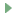# Scientific mode tutorial

In this tutorial, you operate in Scientific Mode and use Matplotlib and NumPy packages to run and debug a Python code with data visualization.

Before you start, make sure that conda is installed.

Create an empty Python project. Add a new Python file named main.py by right-clicking the project root and selecting New | Python File. Then add the following code the hte main.py file:

import numpy as np import matplotlib.pyplot as plt N = 50 x = np.random.rand(N) y = np.random.rand(N) colors = np.random.rand(N) area = np.pi * (15 * np.random.rand(N))**2 # 0 to 15 point radii plt.scatter(x, y, s=area, c=colors, alpha=0.5) plt.show() X = np.linspace(-np.pi, np.pi, 256,endpoint=True) C,S = np.cos(X), np.sin(X) plt.plot(X, C, color="blue", linewidth=2.5, linestyle="-") plt.plot(X, S, color="red", linewidth=2.5, linestyle="-") plt.xlim(X.min()*1.1, X.max()*1.1) plt.xticks([-np.pi, -np.pi/2, 0, np.pi/2, np.pi], [r'$-\pi$', r'$-\pi/2$', r'$0$', r'$+\pi/2$', r'$+\pi$']) plt.ylim(C.min()*1.1,C.max()*1.1) plt.yticks([-1, 0, +1], [r'$-1$', r'$0$', r'$+1$']) plt.show()

Process warnings shown for the numpy and matplotlib imports and enable the packages in the project.

## Running

Ensure that the Scientific mode is enabled (View | Scientific Mode).

Run your scientific project . The code is executed and shows two graphs in the SciView. Clicking the preview thumbnail displays the respective graph:

You can modify the project code to plot only one graph at a time. In the scientific mode, you can execute fragments of your code by creating code cells.

### To execute code cells:

1. Modify the main.py file by adding the "#%%" lines.

2. In the gutter, click the iconon line with the scatter plot cell mark. Only the scatter graph will be built.

3. Now click the iconon the line with the y versus x plot cell mark. The corresponding graph should appear.

## Debugging

Let's put a breakpoint at the line:

plt.show()

This line appears twice in our example code, and so there will be two breakpoints.

Right-click the editor background and from the context menu choose Debug 'main'.

You see the Debug tool window and the grey characters in the editor. This is the result of the inline debugging, which is enabled.

The line with the first breakpoint is blue highlighted. It means that the debugger has stopped at the line with the breakpoint, but has not yet executed it. If we execute this line (for example, by clicking thebutton on the stepping toolbar of the Debug tool window), we'll see the graph:

Next, look at the Variables tab of the Debug tool window. If you click the View as Array link nearby the area array, the Data tab in the SciView window opens:

Mind the only row of figures in the Data tab in the SciView - it's explained by the fact that the area array is one-dimensional.

## Running in console

Right-click the editor background and choose the Run File in Console command:

This command corresponds to running a run/debug configuration for the main.py file with the Run with Python console checkbox selected:

When this command is run, the >>> prompt appears after the output in the Run tool window, and you can execute your own commands.

## Summary

So, what has been done with the help of IntelliJ IDEA?

• The file main.py was created and opened for editing.

• The source code has been entered

• The source code has been run and debugged.

• Finally, we ran the file in console.